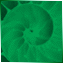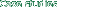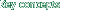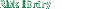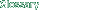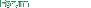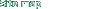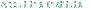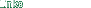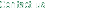Risk Library
 Documents by Author
 International Organization of Securities...
 Methodologies for Determining Capital St...Appendix - Selected VaR Papers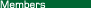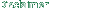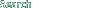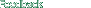### Appendix - Selected VaR Papers

 This appendix does not give a full literature survey of all VaR-related research. Rather, it summarises a selection of papers that have specifically looked at the empirical performance of different VaR models in various circumstances. The summaries are IOSCO's and have not been reviewed by the papers' authors. Further relevant papers may be found in the compilations of VaR-related articles published by Risk Publications (VAR, Understanding and Applying Value at Risk, September 1997) and in the Journal of Derivatives (Spring 1997). Alexander and Leigh. Journal of Derivatives, Spring 1997. 'On the covariance Matrices used in Value at Risk Models'. Generated covariance matrices and tested the performance of different parametric models on equity indices and US\$ exchange rates. Found that an exponentially-weighted parametric model would in many cases be classed as 'Red Zone' under the Basle backtesting framework - although for US equities this type of model performed best in the operational evaluation. Darryl Hendricks, FRBNY Economic policy review, April 1996. 'Evaluation of Value-at-Risk Models using Historical Data'. Applied different types of VaR model, using different data window lengths and different confidence levels, to 1000 randomly chosen FX portfolios. Concluded that in almost all cases the approaches cover the risk they are intended to cover; that the approaches produce risk estimates that do not differ greatly in average size; but that at a 99% confidence level, only a long-window historical simulation approach covered 99% of actual outcomes, with other approaches tending to cover around 98.2-98.5% of outcomes; that VaRs generated by these other approaches would need to be increased by 10-15% to achieve perfect 99% coverage, and that outcomes which lie in the 1% tail are typically 30-40% bigger than the model's VaR. Jackson, Maude & Perraudin, Journal of Derivatives, Spring 1997. 'Bank Capital and Value at Risk'. Examined the performance of different VaR models (with different data window lengths and holding periods) using actual fixed income, FX and equity security portfolios of a large bank. Found that simulation based VaR techniques yielded more accurate measures of tail probabilities than the parametric approaches, where actual losses exceeded the model's 99% cut off much more than 1% of the time. A longer data window did help to reduce the tail probability bias, whereas weighting schemes (in which more recent returns are given a higher weighting than the more lagged ones) tended to increase it. Comparing the model-generated 99% capital with actual losses, the historical simulation approach tended to produce a capital surplus more often and in larger amounts. However, if the capital requirement were calculated using the Basle approach of the 60-day average 10-day VaR multiplied by 3, none of the portfolios under any of the approaches would have had a single loss outlier; indeed a multiplier of 2.5 would suffice. James M. Mahoney. Conference paper of September 1996. Explores which method best forecasts 1 day VaRs at a variety of confidence levels. Selects random currency portfolios and random equity portfolios. Concluded that historical simulation was more accurate for randomly chosen portfolios, particularly at higher confidence levels. However, suggested that for intentionally hedged portfolios, VaR estimates may understate the true value at risk. Marshall and Siegel. Journal of Derivatives, Spring 1997. 'Value at Risk: Implementing a Risk Measurement Standard'. Looked at the variation in VaR outputs produced by different users of the same model (Risk Metrics) using the same test portfolios and the same parameters of risk (one-day, 95% confidence). Concluded that certain instruments - generally the more complex kind - gave rise to significant 'implementation risk', i.e. the risk of significant variations in outputs unrelated to the model being used. Differences in the underlying valuation of instruments was sometimes (but not always) the driving factor behind variations. Matt Pritsker, Risk Publications, 1996. 'Evaluating Value-at-Risk Methodologies: Accuracy Versus Computational Time'. This paper examines six VaR methods, and compares their computational requirement and their accuracy when the sole source of inaccuracy is errors in approximating non-linearity. Simulations using portfolios of foreign exchange options show fairly wide variation in accuracy and unsurprisingly wide variation in computational time. The paper also presents a method for using order statistics to create confidence intervals for the errors and as a percentage of the true value at risk for each VaR method. Tanya Styblo Beder. Financial Analysts Journal. September / October 1995. 'VAR: Seductive but Dangerous'. Applied 8 VaR calculations to 3 hypothetical portfolios. Used historical simulation with two different data bases and two holding periods (1 day and two weeks), and Monte Carlo simulation with two sets of correlation estimates and the same two holding periods. Also applied two confidence levels (95% and 99%). At the extreme, the resulting estimates of VaRs differed by up to 14 times. However, where the parameters were constrained, the differences were much less significant.